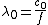# Wavelength measurement

## Wavelength measurement

Determination of the distance between successive wavefronts of equal phase of a wave. See Electromagnetic radiation, Wave motion, Wavelength

From the relation λ = c/f between wavelength λ, speed c, and frequency f, the wavelength of a wave motion can be calculated if the speed is known and its frequency is measured. The ease and accuracy of electronic counting and timing make frequency measurement the most precise of all physical measurements. This method of wavelength determination is thus one of the most accurate, but only if the speed (phase velocity) is known. In free space the speed of an electromagnetic wave c0 is, through the 1983 definition of the meter, fixed at exactly 299,792,458 m/s (186,282.397 mi/s), or roughly 300,000 km/s. Unless otherwise specified, it is general practice to quote the wavelength of an electromagnetic wave as the free-space value λ0, given by the equation below. ()See Frequency measurement, Phase velocity

#### Radio and microwave regions

The presence of any dielectric material (such as air) or any magnetic matter with a permeability greater then unity will cause the wave to travel at a velocity lower than its free-space value. The speed is also altered if the waves pass through an aperture, are focused by a lens or mirror, or are constrained by a wave-guide or transmission line. In such cases it may be more appropriate to measure the wavelength directly. In the pioneering experiments on radio waves, it was found that standing waves existed in space whenever reflections occurred and that these provided a convenient means of measuring the wavelength. It thus became the convention to characterize waves by their wavelength, rather than by their frequency as is now more commonly the case. Specifying the frequency is preferred because, unlike the wavelength, it is independent of the speed of propagation and does not change as the wave moves from one medium to another.

In the microwave region the wavelengths are sufficiently short that it is convenient to measure them by using interferometer techniques directly analogous to those used with light.

In a typical interferometer used in the millimeter wavelength range (see illustration), a microwave beam is directed at a beam splitter, which splits the beam into two parts, A and B, by partial reflection. The A beam is reflected to a movable reflector and reflected again as A. The beam splitter transmits part of this as A. The transmitted part B of the original beam is reflected by a fixed microwave reflector as B. This is partially reflected by the beam splitter as B. The beams A and B combine to form standing waves, which are then detected. Movement of the movable reflector causes the position of the standing wave to move, which causes the detected signal to pass through successive points of maximum and minimum amplitude. The distance between points of successive maxima or minima is one-half wavelength. This distance may be determined from the motion of the movable reflector. See Interferometry

#### Infrared and optical regions

The free-space wavelengths of monochromatic visible and infrared radiations can be derived directly, if their corresponding frequencies are known. The wavelengths of a few stabilized laser radiations have been precisely determined in this way, by reference to the cesium-133 primary frequency standard. These wavelength standards, with uncertainties between 4.5 × 10-10 and 3 × 10-12, are used particularly for the practical realization of the unit of length, but they also serve as reference standards for interferometric wavelength measurements and for the calibration of wavelength comparators based on dispersion methods. See Length, Light, Physical measurement, Wavelength standards

Wavelength values to an accuracy of 1 part in 105 can be determined with a spectrometer, spectrograph, or monochromator, in which a prism or diffraction grating is used as a dispersive element. Each wavelength forms a line image of the entrance slit at a particular angle. An unknown wavelength can be determined by interpolation with the pattern formed by a lamp emitting the tabulated characteristic wavelengths of a particular element. See Diffraction grating, Optical prism, Spectroscopy

The most precise wavelength measurements use an interferometer to compare the unknown wavelength λ1 with a standard wavelength λ2. Usually either the two-beam Michelson form or the multiple-beam Fabry-Perot form of interferometer is used.

When a number of wavelengths are mixed together in the input to a moving-carriage two-beam interferometer, the output signal is the summation of the many separate sine-wave signals having different periods. A Fourier analysis of this composite signal enables the separate wavelengths to be identified. This Fourier transform method is particularly useful for the measurement of complex spectra in the infrared. See Infrared spectroscopy

Mentioned in ?
References in periodicals archive ?
as single wavelength measurement (for protein and dna)
Customer-designed and new application-ready preconfigured NanoCalc models make it easy to select the optimum system for deep UV to NIR wavelength measurement needs.
In the case of DE measurement, quantification involves more than a single wavelength measurement.
41 * the uncertainty of the wavelength measurement is of the order of 0.
The Tektronix OTS9600 Series of optical test modules is a swept wavelength measurement system that addresses physical-layer testing for passive optical components.
The user can work in Absorbance or % Transmission, and the instrument provides single wavelength measurement or instant spectral scans.
The center wavelength measurement had a range of about 27 pm in the sensors group and is not adequate to support long-term structural monitoring applications.
It performs single wavelength measurements of absorbance, % transmission, concentration and has the ability to perform simple kinetics.

Site: Follow: Share:
Open / Close